# Dowhy: An end-to-end library for causal inference

Nov. 11, 2020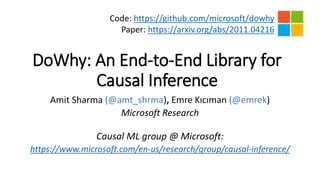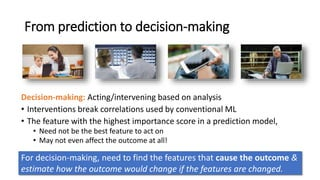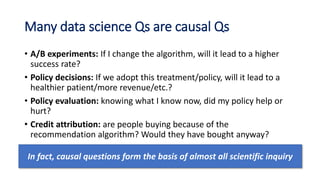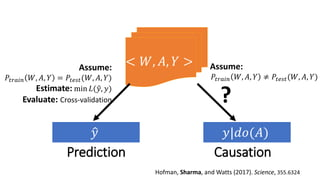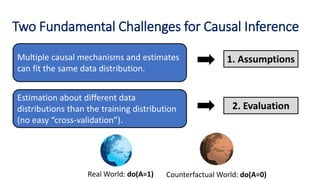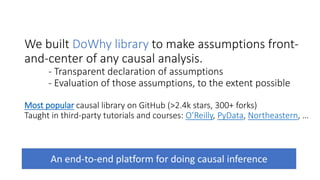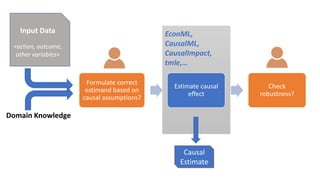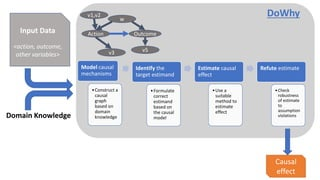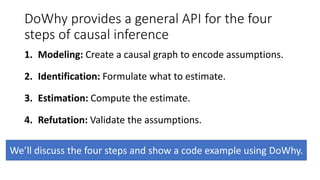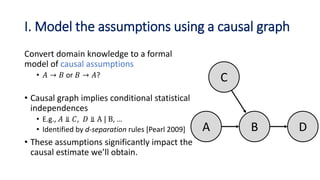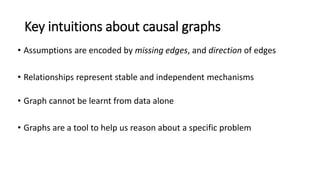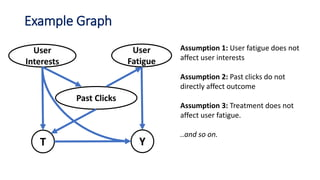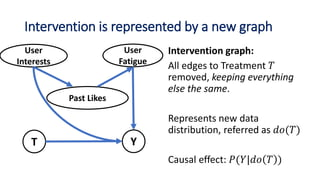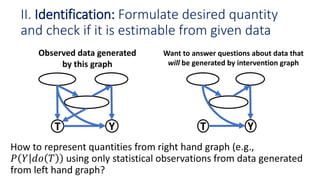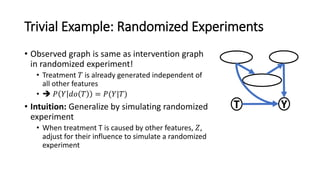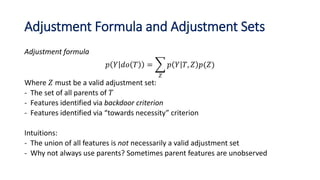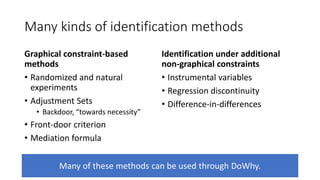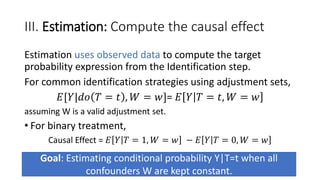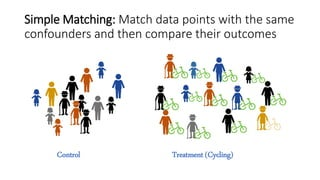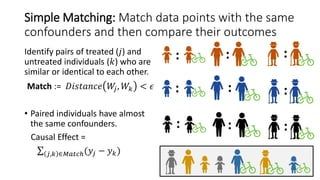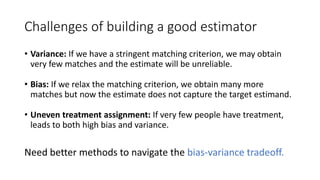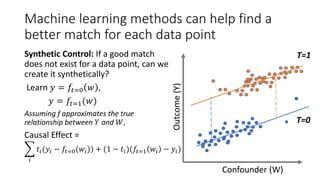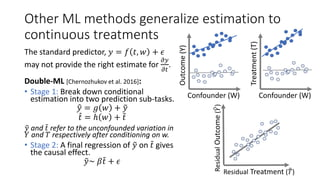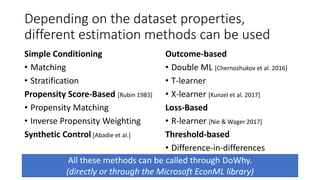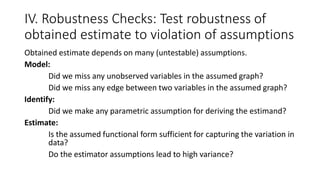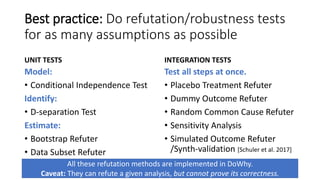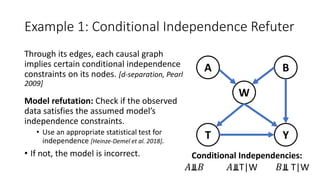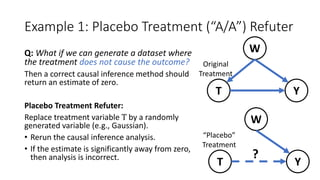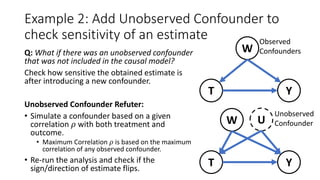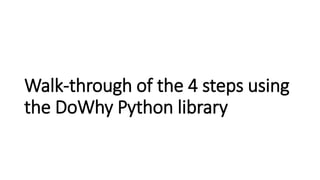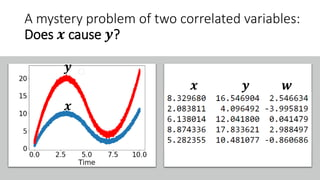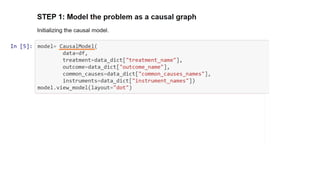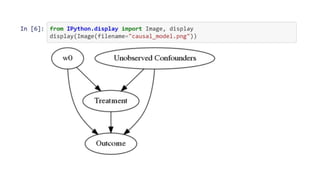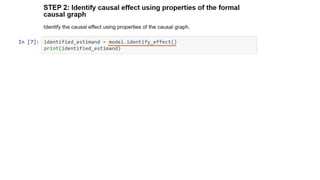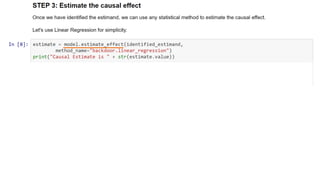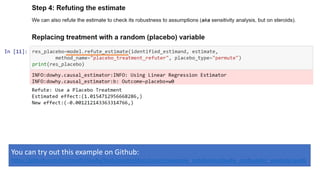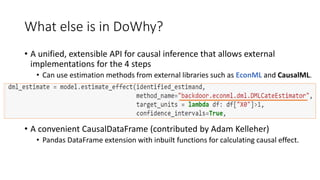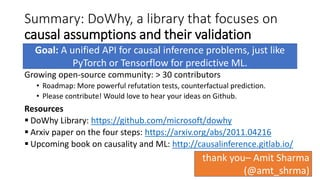1 of 38

### Dowhy: An end-to-end library for causal inference

1. DoWhy: An End-to-End Library for Causal Inference Amit Sharma (@amt_shrma), Emre Kıcıman (@emrek) Microsoft Research Causal ML group @ Microsoft: https://www.microsoft.com/en-us/research/group/causal-inference/ Code: https://github.com/microsoft/dowhy Paper: https://arxiv.org/abs/2011.04216
2. From prediction to decision-making Decision-making: Acting/intervening based on analysis • Interventions break correlations used by conventional ML • The feature with the highest importance score in a prediction model, • Need not be the best feature to act on • May not even affect the outcome at all! For decision-making, need to find the features that cause the outcome & estimate how the outcome would change if the features are changed.
3. Many data science Qs are causal Qs • A/B experiments: If I change the algorithm, will it lead to a higher success rate? • Policy decisions: If we adopt this treatment/policy, will it lead to a healthier patient/more revenue/etc.? • Policy evaluation: knowing what I know now, did my policy help or hurt? • Credit attribution: are people buying because of the recommendation algorithm? Would they have bought anyway? In fact, causal questions form the basis of almost all scientific inquiry
4. Prediction Causation Assume: 𝑃𝑡𝑟𝑎𝑖𝑛 𝑊, 𝐴, 𝑌 = 𝑃𝑡𝑒𝑠𝑡(𝑊, 𝐴, 𝑌) Estimate: min 𝐿( 𝑦, 𝑦) Evaluate: Cross-validation Assume: 𝑃𝑡𝑟𝑎𝑖𝑛 𝑊, 𝐴, 𝑌 ≠ 𝑃𝑡𝑒𝑠𝑡(𝑊, 𝐴, 𝑌)
5. Two Fundamental Challenges for Causal Inference Multiple causal mechanisms and estimates can fit the same data distribution. Estimation about different data distributions than the training distribution (no easy “cross-validation”). 1. Assumptions 2. Evaluation Real World: do(A=1) Counterfactual World: do(A=0)
6. We built DoWhy library to make assumptions front- and-center of any causal analysis. - Transparent declaration of assumptions - Evaluation of those assumptions, to the extent possible Most popular causal library on GitHub (>2.4k stars, 300+ forks) Taught in third-party tutorials and courses: O’Reilly, PyData, Northeastern, … An end-to-end platform for doing causal inference
7. EconML, CausalML, CausalImpact, tmle,… Formulate correct estimand based on causal assumptions? Estimate causal effect Check robustness? Input Data <action, outcome, other variables> Domain Knowledge Causal Estimate
8. Model causal mechanisms •Construct a causal graph based on domain knowledge Identify the target estimand •Formulate correct estimand based on the causal model Estimate causal effect •Use a suitable method to estimate effect Refute estimate •Check robustness of estimate to assumption violations Input Data <action, outcome, other variables> Domain Knowledge Causal effect DoWhy Action w Outcome v3 v5 v1,v2
9. DoWhy provides a general API for the four steps of causal inference 1. Modeling: Create a causal graph to encode assumptions. 2. Identification: Formulate what to estimate. 3. Estimation: Compute the estimate. 4. Refutation: Validate the assumptions. We’ll discuss the four steps and show a code example using DoWhy.
10. I. Model the assumptions using a causal graph Convert domain knowledge to a formal model of causal assumptions • 𝐴 → 𝐵 or 𝐵 → 𝐴? • Causal graph implies conditional statistical independences • E.g., 𝐴 ⫫ 𝐶, 𝐷 ⫫ A | B, … • Identified by d-separation rules [Pearl 2009] • These assumptions significantly impact the causal estimate we’ll obtain. A C B D
11. Key intuitions about causal graphs • Assumptions are encoded by missing edges, and direction of edges • Relationships represent stable and independent mechanisms • Graph cannot be learnt from data alone • Graphs are a tool to help us reason about a specific problem
12. Example Graph Assumption 1: User fatigue does not affect user interests Assumption 2: Past clicks do not directly affect outcome Assumption 3: Treatment does not affect user fatigue. ..and so on. User Interests YT User Fatigue Past Clicks
13. Intervention is represented by a new graph User Interests YT User Fatigue Past Likes
14. YTYT Want to answer questions about data that will be generated by intervention graph Observed data generated by this graph II. Identification: Formulate desired quantity and check if it is estimable from given data
15. Trivial Example: Randomized Experiments • Observed graph is same as intervention graph in randomized experiment! • Treatment 𝑇 is already generated independent of all other features •  𝑃 𝑌 𝑑𝑜 𝑇 = 𝑃(𝑌|𝑇) • Intuition: Generalize by simulating randomized experiment • When treatment T is caused by other features, 𝑍, adjust for their influence to simulate a randomized experiment YT
16. Adjustment Formula and Adjustment Sets Adjustment formula 𝑝 𝑌 𝑑𝑜 𝑇 = 𝑍 𝑝 𝑌 𝑇, 𝑍 𝑝(𝑍) Where 𝑍 must be a valid adjustment set: - The set of all parents of 𝑇 - Features identified via backdoor criterion - Features identified via “towards necessity” criterion Intuitions: - The union of all features is not necessarily a valid adjustment set - Why not always use parents? Sometimes parent features are unobserved
17. Many kinds of identification methods Graphical constraint-based methods • Randomized and natural experiments • Adjustment Sets • Backdoor, “towards necessity” • Front-door criterion • Mediation formula Identification under additional non-graphical constraints • Instrumental variables • Regression discontinuity • Difference-in-differences Many of these methods can be used through DoWhy.
18. III. Estimation: Compute the causal effect Estimation uses observed data to compute the target probability expression from the Identification step. For common identification strategies using adjustment sets, 𝐸[𝑌|𝑑𝑜 𝑇 = 𝑡 , 𝑊 = 𝑤]= 𝐸 𝑌 𝑇 = 𝑡, 𝑊 = 𝑤 assuming W is a valid adjustment set. • For binary treatment, Causal Effect = 𝐸 𝑌 𝑇 = 1, 𝑊 = 𝑤 − 𝐸 𝑌 𝑇 = 0, 𝑊 = 𝑤 Goal: Estimating conditional probability Y|T=t when all confounders W are kept constant.
19. Control Treatment (Cycling) Simple Matching: Match data points with the same confounders and then compare their outcomes
20. Simple Matching: Match data points with the same confounders and then compare their outcomes Identify pairs of treated (𝑗) and untreated individuals (𝑘) who are similar or identical to each other. Match := 𝐷𝑖𝑠𝑡𝑎𝑛𝑐𝑒 𝑊𝑗, 𝑊𝑘 < 𝜖 • Paired individuals have almost the same confounders. Causal Effect = 𝑗,𝑘 ∈𝑀𝑎𝑡𝑐ℎ(𝑦𝑗 − 𝑦 𝑘) : : : : : : : : :
21. Challenges of building a good estimator • Variance: If we have a stringent matching criterion, we may obtain very few matches and the estimate will be unreliable. • Bias: If we relax the matching criterion, we obtain many more matches but now the estimate does not capture the target estimand. • Uneven treatment assignment: If very few people have treatment, leads to both high bias and variance. Need better methods to navigate the bias-variance tradeoff.
22. Machine learning methods can help find a better match for each data point Synthetic Control: If a good match does not exist for a data point, can we create it synthetically? Learn 𝑦 = 𝑓𝑡=0 𝑤 , 𝑦 = 𝑓𝑡=1(𝑤) Assuming f approximates the true relationship between 𝑌 and 𝑊, Causal Effect = 𝑖 𝑡𝑖(𝑦𝑖 − 𝑓𝑡=0(𝑤𝑖)) + (1 − 𝑡𝑖)(𝑓𝑡=1 𝑤𝑖 − 𝑦𝑖) Confounder (W) Outcome(Y) Confounder T=1 T=0
23. Other ML methods generalize estimation to continuous treatments The standard predictor, 𝑦 = 𝑓 𝑡, 𝑤 + 𝜖 may not provide the right estimate for 𝜕𝑦 𝜕𝑡 . Double-ML [Chernozhukov et al. 2016]: • Stage 1: Break down conditional estimation into two prediction sub-tasks. 𝑦 = 𝑔 𝑤 + 𝑦 𝑡 = ℎ 𝑤 + 𝑡 𝑦 and 𝑡 refer to the unconfounded variation in 𝑌 and 𝑇 respectively after conditioning on w. • Stage 2: A final regression of 𝑦 on 𝑡 gives the causal effect. 𝑦~ 𝛽 𝑡 + 𝜖 Outcome(Y) Confounder (W) Treatment(T) Confounder (W) Residual Treatment ( 𝑇) ResidualOutcome(𝑌)
24. Depending on the dataset properties, different estimation methods can be used Simple Conditioning • Matching • Stratification Propensity Score-Based [Rubin 1983] • Propensity Matching • Inverse Propensity Weighting Synthetic Control [Abadie et al.] Outcome-based • Double ML [Chernozhukov et al. 2016] • T-learner • X-learner [Kunzel et al. 2017] Loss-Based • R-learner [Nie & Wager 2017] Threshold-based • Difference-in-differences All these methods can be called through DoWhy. (directly or through the Microsoft EconML library)
25. IV. Robustness Checks: Test robustness of obtained estimate to violation of assumptions Obtained estimate depends on many (untestable) assumptions. Model: Did we miss any unobserved variables in the assumed graph? Did we miss any edge between two variables in the assumed graph? Identify: Did we make any parametric assumption for deriving the estimand? Estimate: Is the assumed functional form sufficient for capturing the variation in data? Do the estimator assumptions lead to high variance?
26. Best practice: Do refutation/robustness tests for as many assumptions as possible UNIT TESTS Model: • Conditional Independence Test Identify: • D-separation Test Estimate: • Bootstrap Refuter • Data Subset Refuter INTEGRATION TESTS Test all steps at once. • Placebo Treatment Refuter • Dummy Outcome Refuter • Random Common Cause Refuter • Sensitivity Analysis • Simulated Outcome Refuter /Synth-validation [Schuler et al. 2017] All these refutation methods are implemented in DoWhy. Caveat: They can refute a given analysis, but cannot prove its correctness.
27. Example 1: Conditional Independence Refuter Through its edges, each causal graph implies certain conditional independence constraints on its nodes. [d-separation, Pearl 2009] Model refutation: Check if the observed data satisfies the assumed model’s independence constraints. • Use an appropriate statistical test for independence [Heinze-Demel et al. 2018]. • If not, the model is incorrect. W YT A B Conditional Independencies: 𝐴⫫𝐵 𝐴⫫T|W 𝐵⫫ T|W
28. Example 1: Placebo Treatment (“A/A”) Refuter Q: What if we can generate a dataset where the treatment does not cause the outcome? Then a correct causal inference method should return an estimate of zero. Placebo Treatment Refuter: Replace treatment variable T by a randomly generated variable (e.g., Gaussian). • Rerun the causal inference analysis. • If the estimate is significantly away from zero, then analysis is incorrect. W YT W YT ? Original Treatment “Placebo” Treatment
29. Example 2: Add Unobserved Confounder to check sensitivity of an estimate Q: What if there was an unobserved confounder that was not included in the causal model? Check how sensitive the obtained estimate is after introducing a new confounder. Unobserved Confounder Refuter: • Simulate a confounder based on a given correlation 𝜌 with both treatment and outcome. • Maximum Correlation 𝜌 is based on the maximum correlation of any observed confounder. • Re-run the analysis and check if the sign/direction of estimate flips. W YT Observed Confounders W YT U Unobserved Confounder
30. Walk-through of the 4 steps using the DoWhy Python library
31. 𝒙 𝒚 𝒙 𝒚 𝒘 A mystery problem of two correlated variables: Does 𝒙 cause 𝒚?
32. You can try out this example on Github: https://github.com/microsoft/dowhy/blob/master/docs/source/example_notebooks/dowhy_confounder_example.ipynb
33. What else is in DoWhy? • A unified, extensible API for causal inference that allows external implementations for the 4 steps • Can use estimation methods from external libraries such as EconML and CausalML. • A convenient CausalDataFrame (contributed by Adam Kelleher) • Pandas DataFrame extension with inbuilt functions for calculating causal effect.
34. Summary: DoWhy, a library that focuses on causal assumptions and their validation Growing open-source community: > 30 contributors • Roadmap: More powerful refutation tests, counterfactual prediction. • Please contribute! Would love to hear your ideas on Github. Resources  DoWhy Library: https://github.com/microsoft/dowhy  Arxiv paper on the four steps: https://arxiv.org/abs/2011.04216  Upcoming book on causality and ML: http://causalinference.gitlab.io/ Goal: A unified API for causal inference problems, just like PyTorch or Tensorflow for predictive ML. thank you– Amit Sharma (@amt_shrma)